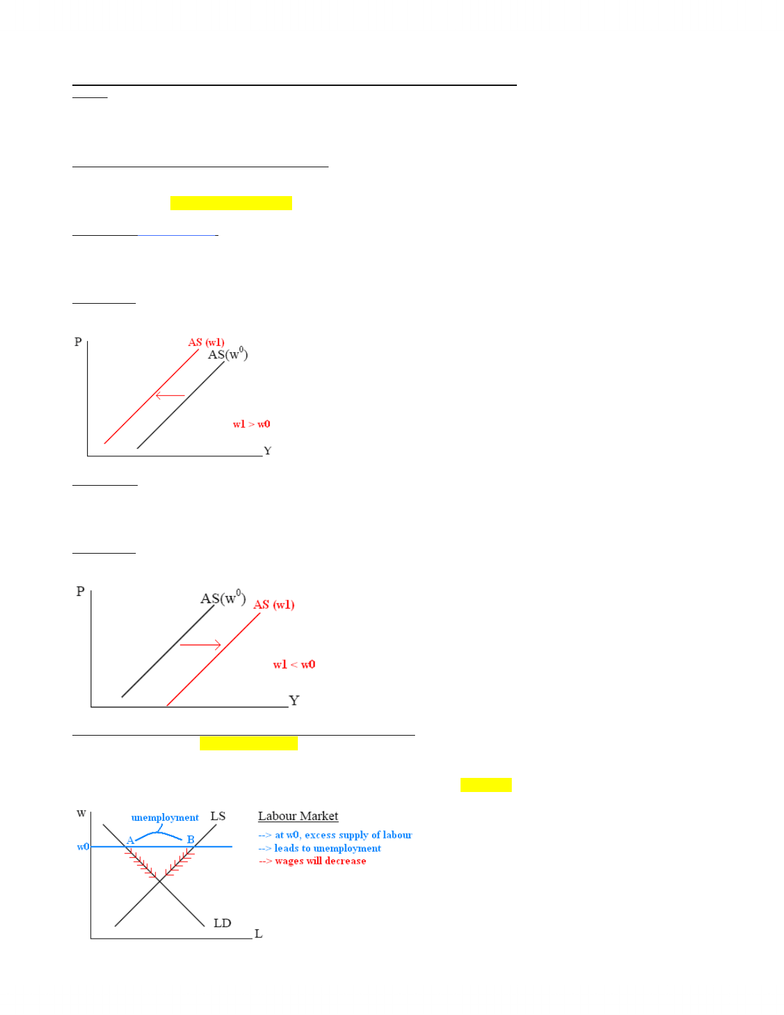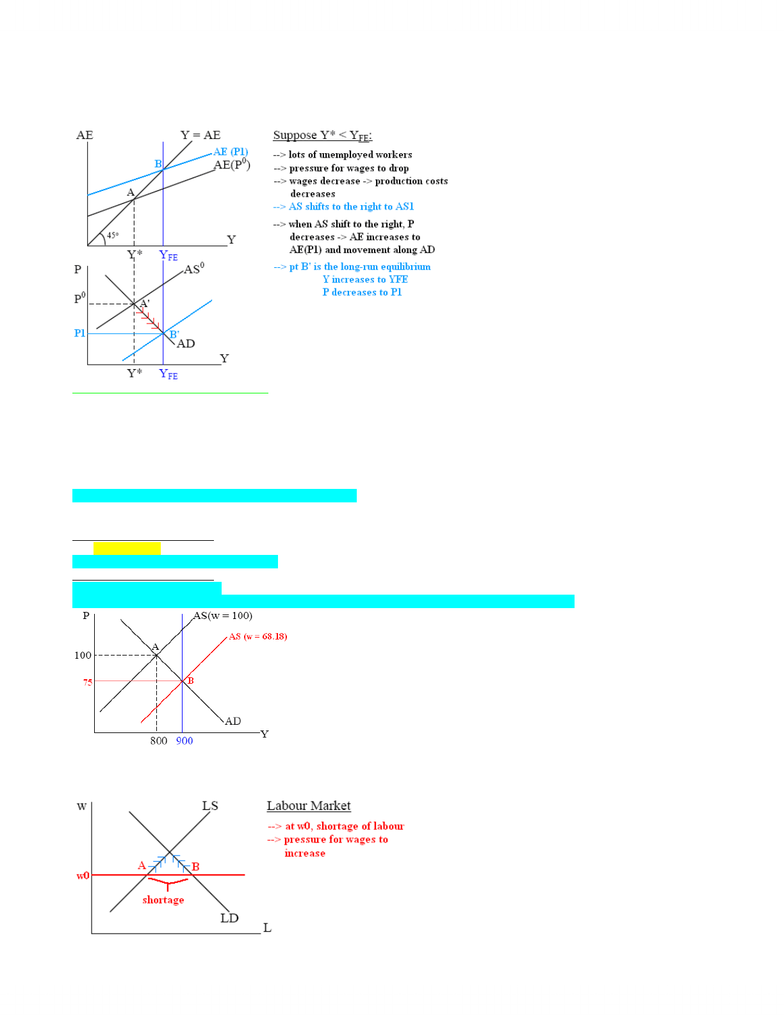# Lecture notes for week 7

33 views6 pagesTHE AS-AD MODEL IN THE LONG RUN & BRINGING MONEY INTO THE MODEL
Outline
x Discuss the adjustment mechanism from the short run to the long run.
x Discuss how the economy will correct itself to its long-run equilibrium if Y in the short run (Y*) YFE.
x Introducing money in our model.
The Effect of a Change in Wages on AS and AD Curves
x The question we ask: Is there a natural adjustment mechanism that can eliminate inflationary or deflationary gap in the long run?
x The theory says YES, inflationary or deflationary gap can be eliminated by change in (nominal) wages in the long run.
x Question: How changes in nominal wages affect the AD and AS curve?
Case 1: A Rise in (Nominal) Wages
Effect on AD (setting AE = Y):
AE = C (Y – T + TR) + I (r) + G + X (E) – IM (E, Y)
x Since wages do not enter the AE function, a change in wages would have no effect on AE Æ No effect on AD.
x Note: A rise in wages raises workers’ income, but it lowers shareholders’ income (profit falls) Æ overall no change in (total) real
income Æ no change in AE Æ AD does not shift when wages increase.
Effect on AS:
x When wages rise, production costs increases Æ firms profit decreases.
x For any given price level, firms’ profit decreases Æ firms’ willingness to supply decreases Æ AS curve shifts to the left.
Case 2: A Fall in (Nominal) Wages
AE = C (Y – T + TR) + I (r) + G + X (E) – IM (E, Y)
x Since wages do not enter the AE function, a change in wages would have no effect on AE Æ No effect on AD.
x Note: A fall in wages lowers workers’ income, but in raises shareholders’ income (profit rise) Æ overall no change in (total) real
income Æ no change in AE Æ AD does not shift.
Effect on AS:
x When wages fall, production costs decreases Æ firms’ profit increases.
x For any given price level, firms’ profit increases Æ firms’ willingness to supply increases Æ AS curve shifts to the right.
The (Natural) Adjustment Mechanism from the Short Run to the Long Run
x We argue that there is pressure for wages to change if Y* does not equal YFE in the short run.
x Question: What is the actual adjustment mechanism if there is a deflationary gap? An inflationary gap?
Case 1: Adjustment Mechanism in a Deflationary Gap
x Question: What happens to wages in the long run if there is a deflationary gap (Y* < YFE)?
x Answer: Since there is lots of unemployment (the initial wage is too high), there will be pressure for wages to fall.
www.notesolution.com
Unlock document

This preview shows pages 1-2 of the document.
Unlock all 6 pages and 3 million more documents.x Question: Why there is pressure for wages to fall?
x Answer: At w0, there is excess supply of labour.
o Unemployed workers offer lower wages in order to get a job.
o Firms should pressure existing workers to accept lower wage because of plenty alternate workers.
Numerical Example—A Deflationary Gap
The AS-AD model with a flat AS curve (a deflationary gap):
AS: P = (20 + 0.1Y) (w / 100), initial wage (w0) = 100
x Note: We modify the AS function slightly to include wages. The rationale is wages are a major cost of production, a rise in wages
requires a rise in price if firms are to supply the same level of Y.
x Initial short-run equilibrium:
Y* = 800 P = 100
Æ
20 + 0.1Y = 300 – ¼ Y = Y* = 800
Now, suppose the full-employment level of output is 900 (i.e., YFE = 900).
How does the economy adjust itself back to YFE? What happens to the price and wages in the long run?
Step 1: Solving for P from AD
Sub Y = YFE = 900 into AD:
P = 300¼ (900) = 75 (P decreases by 25)
Step 2: Solving for w from AS
Sub Y = 900 and P = 75 into AS
75 = (20 + 0.1 (900)) (w /100)
Æ
7500 = (20 + 90) w
Æ
7500 = 110w
Æ
w = 68.18 (w decreases by 31.82)
Case 2: Adjustment Mechanism in an Inflationary Gap
x Question: What happens to wages in the long run if there is an inflationary gap (Y* > YFE)?
x Answer: The labour market is tight (wages set too low and there is excess demand for labour); there is pressure for wages to rise
(firms compete to grab each other good workers, good workers try to look for better paying jobs).
www.notesolution.com
Unlock document

This preview shows pages 1-2 of the document.
Unlock all 6 pages and 3 million more documents.

## Document Summary

The as-ad model in the long run & bringing money into the model. N discuss the adjustment mechanism from the short run to the long run. N discuss how the economy will correct itself to its long-run equilibrium if y in the short run (y*) yfe. The effect of a change in wages on as and ad curves. N note: a rise in wages raises workers" income, but it lowers shareholders" income (profit falls) overall no change in (total) real. The theory says yes, inflationary or deflationary gap can be eliminated by change in (nominal) wages in the long run. Ae = c (y t + tr) + i (r) + g + x (e) im (e, y) income no change in ae ad does not shift when wages increase. N when wages rise, production costs increases firms" profit decreases.

## Get access

\$10 USD/m
Billed \$120 USD annuallyHomework Help
Study Guides
Textbook Solutions
Class Notes
Textbook Notes
Booster Class